Tamilnadu State Board New Syllabus Samacheer Kalvi 11th Maths Guide Pdf Chapter 5 Binomial Theorem, Sequences and Series Ex 5.2 Text Book Back Questions and Answers, Notes.

## Tamilnadu Samacheer Kalvi 11th Maths Solutions Chapter 5 Binomial Theorem, Sequences and Series Ex 5.2

Question 1.
Write the first 6 terms of the sequence whose nth terms are given below and classify them as Arithmetic progression Geometric progression, Arithmetic – geometric progression, Harmonic progression and none of them.
(i) $$\frac{1}{2^{n+1}}$$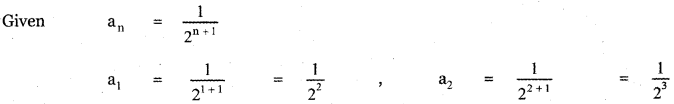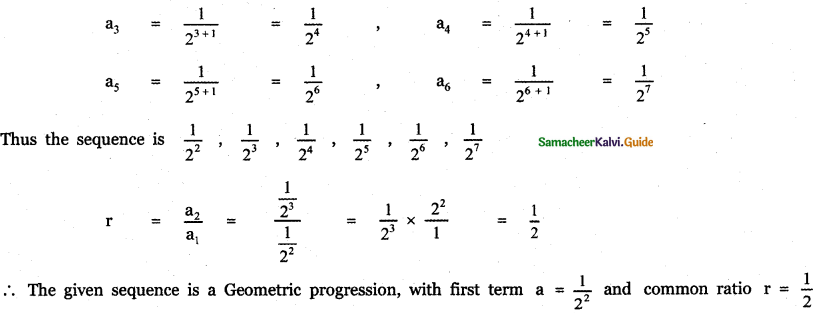(ii)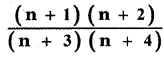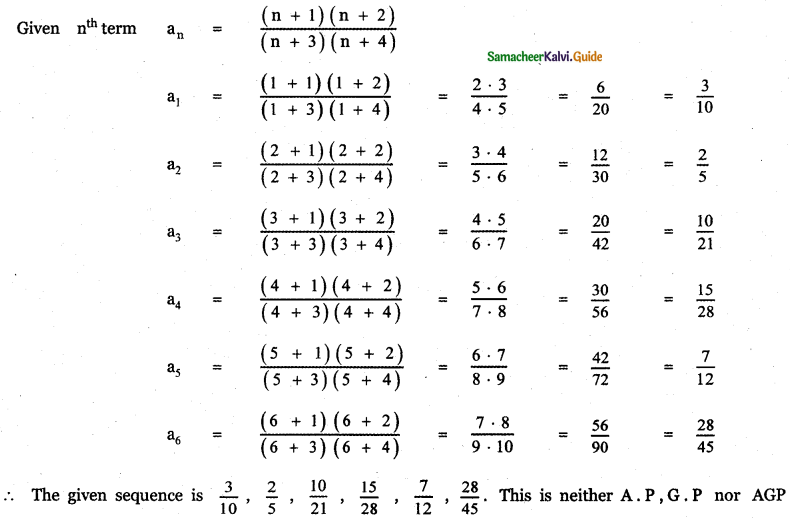(iii) $$4\left(\frac{1}{2}\right)^{n}$$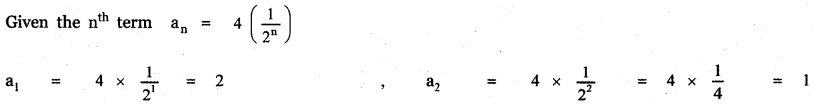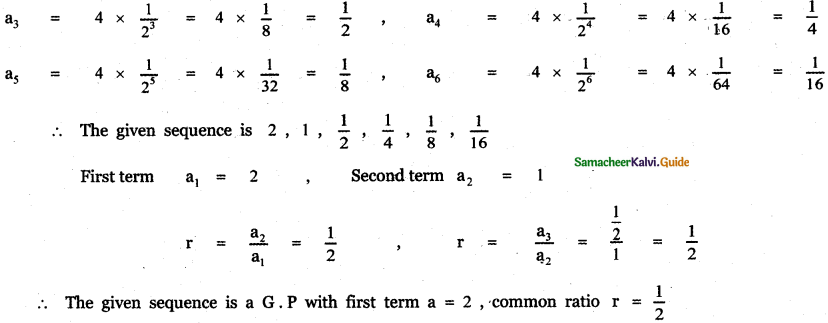(iv) $$\frac{(-1)^{n}}{n}$$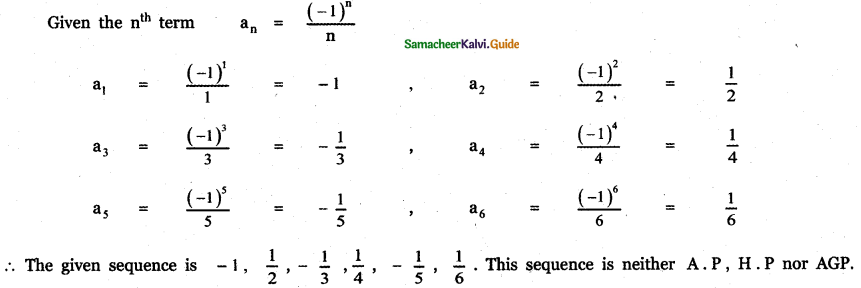(v) $$\frac{2 n+3}{3 n+4}$$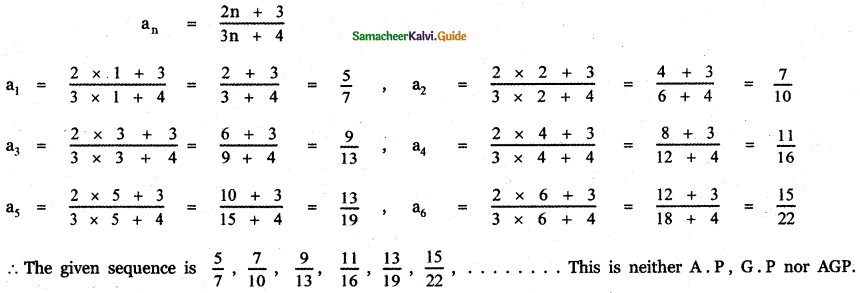(vi) 2018
The nth term an = 2018
a1 = 2018,
a2 = 2018,
a3 = 2018,
a4 = 2018,
a5 = 2018,
a6 = 2018,
∴ The given sequence is 2018, 2018, 2018, 2018, 2018, 2018, ………….
This is a œnstant sequence which has same common ratio and common difference.
Hence this is an A. P, G . P and AGP.

(vii) $$\frac{3 n-2}{3^{n-1}}$$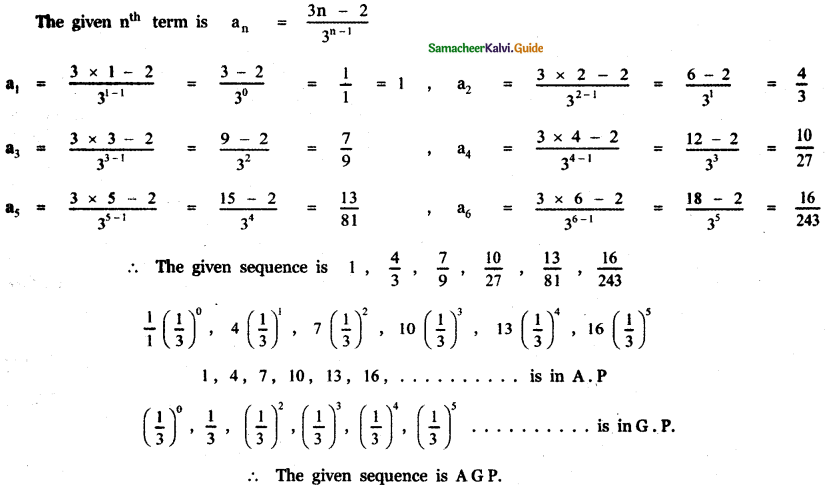Question 2.
Write the first 6 terms of the sequences whose nth term an is given below
(i)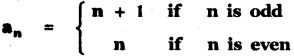n = 1, an = n + 1, a1 = 1 + 1 = 2
n = 2, an = n, a2 = 2
n = 3, an = n + 1, a3 = 3 + 1 = 4
n = 4, an = n, a4 = 4
n = 5, an = n + 1, a5 = 5 + 1 = 6
n = 6, an = n, a6 = 6
∴ The first six terms are 2, 2, 4, 4, 6, 6

(ii)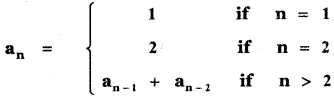n = 1, a1 = 1, n = 2, a2 = 2
n = 3, an = an+1 + an-2, a3 = a3 – 1 + a3 – 2 = a2 + a1 = 2 + 1 = 3

n = 4, an = an+1 + an-2, a4 = a4 – 1 + a4 – 2 = a3 + a2 = 3 + 2 = 5

n = 5, an = an+1 + an-2, a5 = a5 – 1 + a5 – 2 = a4 + a3 = 5 + 3 = 8

n = 6, an = an+1 + an-2, a6 = a6 – 1 + a6 – 2 = a5 + a4 = 8 + 5 = 13
∴ The first six terms are 1, 2, 3, 5, 8, 13

(iii)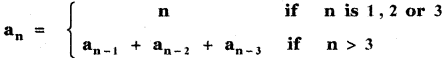n = 1, an = n, a1 = 1
n = 2, an = n, a2 = 1
n = 3, an = n, a3 = 1

n = 4, an = an-1 + an-2 + an-3
a4 = a4-1 + a4-2 + a4-3
a4 = a3 + a2 + a1
a4 = 3 + 2 + 1 = 6

n = 5, an = an-1 + an-2 + an-3
a5 = a5-1 + a5-2 + a5-3
a5 = a4 + a3 + a2
a5 = 6 + 3 + 2 = 11

n = 6, an = an-1 + an-2 + an-3
a6 = a6-1 + a6-2 + a6-3
a6 = a5 + a4 + a3
a6 = 11 + 6 + 3 = 20
∴ The first six terms are 1, 2, 3, 6, 11, 20Question 3.
Write the nth term of the following sequences.
(i) 2, 2, 4, 4, 6, 6, ……………..
The odd terms are a1 = 2, a3 = 4, a5 = 6
The even terms are a2 = 2, a4 = 4, a6 = 6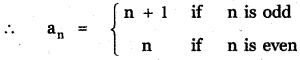(ii)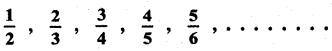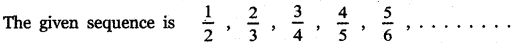The terms in the numerator are 1 , 2 , 3, 4
a = 1 , d = 2 – 1 = 1
an = a + (n – 1) d
an = 1 + (n – 1)(1) = 1 + n – 1 = n
an = n
The terms in the denominator are 2 , 3 , 4 , 5 , 6 .
a = 2, d = 3 – 2 = 1
an = a + (n – 1) d
an = 2 + (n – 1) (1) = 2 + n – 1 = n + 1
an = n + 1
∴ The nth term of the given sequence is an = $$\frac{n}{n+1}$$ for all n ∈ N

(iii)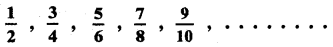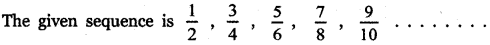The terms in the numerator are 1, 3, 5, 7, 9, ………….
a = 1 , d = 3 – 1 = 2
an = a + (n – 1) d
an = 1 + (n – 1)2
an = 1 + 2n – 2 = 2n – 1
The terms in the denominator are 2, 4, 6, 8, 10, …………..
a = 2, d = 4 – 2 = 2
an = a + (n – 1) d
an = 2 + (n – 1)(2)
an = 2 + 2n – 2 = 2n
∴ The nth term of the given sequence is an = $$\frac{2 n-1}{2 n}$$ for all n ∈ N(iv) 6, 10, 4, 12, 2, 14, 0, 16, – 2 …………………
The given sequence is 6, 10, 4, 12, 2, 14, 0, 16, – 2 ……………..
The odd terms are a1 = 6, a3 = 4 , a5 = 2 , a7 = 0, a9 = – 2
∴ an = n – 7, n is odd
The even terms are a2 = 10, a4 = 12 , a6 = 14 , a8 = 16
∴ an = 8 + n, n is even.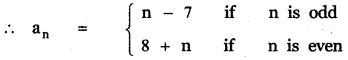Question 4.
The product of three increasing numbers in a G.P is 5832 . If we add 6 to the second number and 9 to the third number, then resulting numbers form an A.P. Find the numbers in G.P.
Let the increasing numbers in G.P be , a, ar.
Given $$\frac{a}{r}$$ × a × ar = 5832 ⇒ a3 = 5832 = 183 ⇒ a = 18
Also given $$\frac{a}{r}$$, a + 6, ar + 9 form an A.P.
∴ 2(a + b) = $$\frac{a}{r}$$ + (ar + 9)
⇒ (a + 6) + (a + 6) = $$\frac{a}{r}$$ + (ar + 9)
⇒ (a + 6) – $$\frac{a}{r}$$ = (ar + 9) – (a + 6)
⇒ a + 6 – $$\frac{a}{r}$$ = ar + 9 – a – 6
⇒ a + 6 – $$\frac{a}{r}$$ = ar – a + 3
Substituting the value of a = 18, we get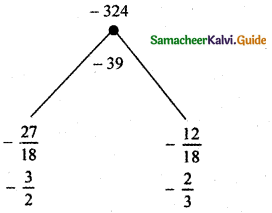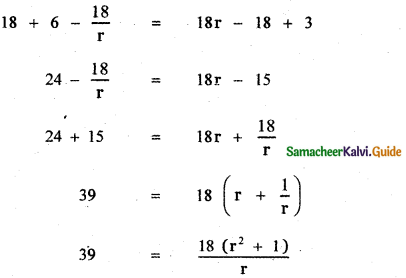39r = 18r2 + 18
18r2 – 39r + 18 = 0
(2r – 3)(3r -2) = 0
2r – 3 = 0 or 3r – 2 = 0
r = $$\frac{3}{2}$$ or r = $$\frac{2}{3}$$Case (i) When a = 18, r = $$\frac{3}{2}$$ the numbers in G.P are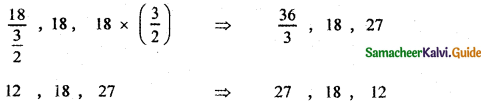Case (ii) When a = 18, r = $$\frac{2}{3}$$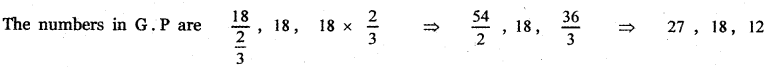Question 5.
Write the nth term of the sequence $$\frac{3}{1^{2} \cdot 2^{2}}, \frac{5}{2^{2} \cdot 3^{2}}, \frac{7}{3^{2} \cdot 4^{2}}$$, …………….. as a difference of two terms.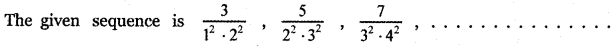The terms in the numerator are 3,5, 7
which forms an A. P with first term a = 3 and common difference d = 5 – 3 = 2
nth term tn = a + (n – 1) d
= 3 + (n – 1)(2)
= 3 + 2n – 2 = 2n + 1
tn = 2n + 1
The terms in the denominator are 12 . 22, 22 . 32, 32 . 42 ……………….
nth term tn = n2 . (n + 1)2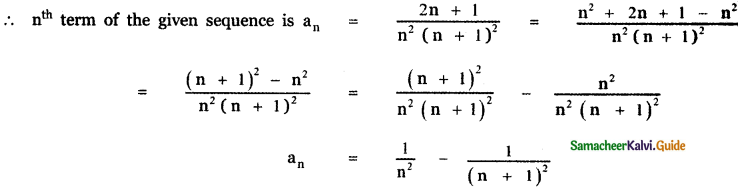Question 6.
If tk is the kth term of a G.P then show that tn – k, tk, tn + k also form a G.F for any positive integer k.
Given tk is the kth term of a G.P. We have nth term of a G.P is tn = arn-1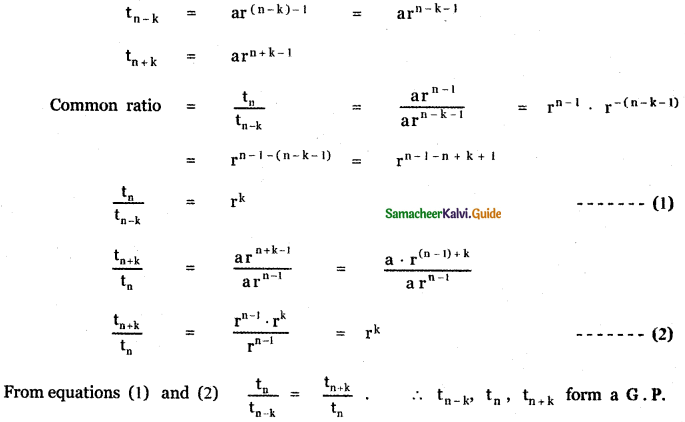Question 7.
If a, b, c are in geometric progression and if a1/x = b1/y = c1/z are in Arithmetic progression.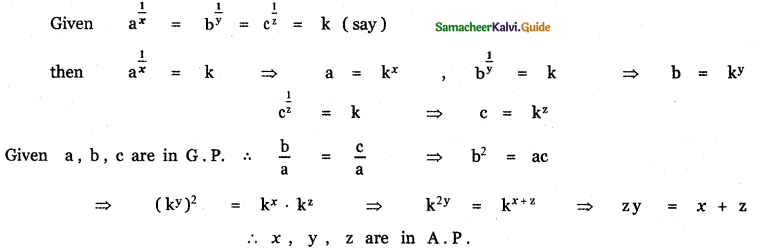Question 8.
The A.M of two numbers exceeds their G.M by 10 and H.M by 16. Find the numbers.
Let the numbers be a and b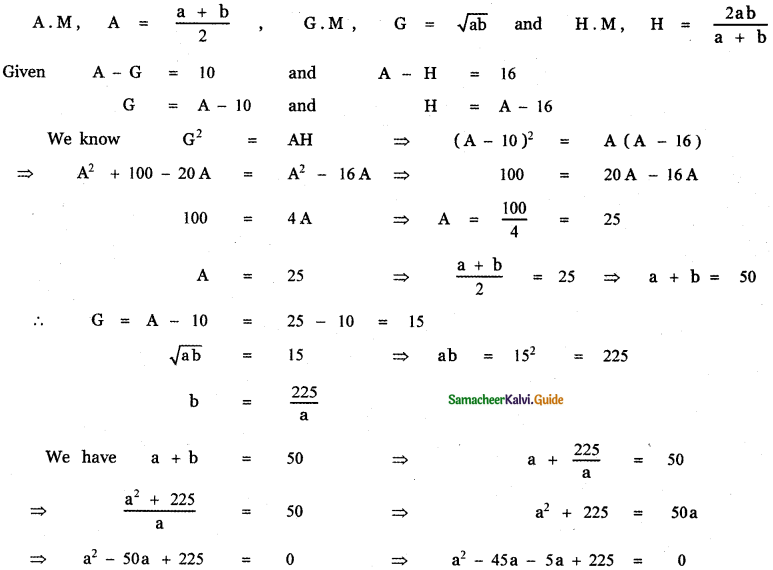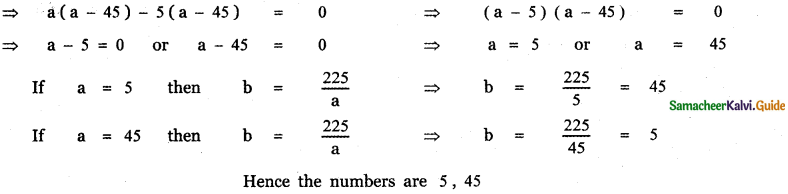Question 9.
If the roots of the equation (q – r)x2 + (r – p)x + (p – q) = 0 are equal then show that p , q and r are in A. P.
The roots are equal ⇒ ∆ = 0
(i.e.) b2 – 4ac = 0
Hence, a = q – r ; b = r – p ; c = p – q
b2 – 4ac = 0
⇒ (r – p)2 – 4(q – r)(p – q) = 0
r2 + p2 – 2pr – 4[qr – q2 – pr + pq] = 0
r2 + p2 – 2pr – 4qr + 4q2 + 4pr – 4pq = 0
(i.e.) p2 + 4q2 + r2 – 4pq – 4qr + 2pr = 0
(i.e.) (p – 2q + r)2 = 0
⇒ p – 2q + r = 0
⇒ p + r = 2q
⇒ p, q, r are in A.P.

Question 10.
If a , b , c are respectively the pth, qth and rth terms of a G . P show that (q – r) log a + (r – p) log b + (p – q) log c = 0
Let A be first term and R be the jmnon ratio of the G.P.
Given a = pth term of the G.P
General term of a G. P with first term A and common ratio R is tn = ARn – 1
∴ a = tp = ARP – 1
log a = log ARp-1 = log A + log Rp-1 = log A + (p – 1) log R

b = qth term of the G.P
b = tq = ARq-1
log b = log ARq-1 = log A + log Rq-1 = log A + (q – r)log R

c = rth term of the G.P
c = tr = ARr-1
log c = log ARr-1 = log A + log Rr-1 = log A + (r – 1) log R(q – r) log a + (r – p) log b + (p – q) log c
= (q – r) [log A + (p – 1) log R] + (r – p) [ log A + (q – 1) log R ] + (P – q) [ log A + (r – 1) log R]
= (q – r) log A + (q – r) (p – 1 ) log R + (r – p) log A + (r – p) (q – 1) log R + (P – q) log A + (p – q) (r – 1 ) log R
= [ q – r + r – p + p – q ] log A + [ (q – r) (p – 1) + (r – p) (q – 1) + (p – q)(r – 1)] log R
= 0 × log A + [pq – q – rp + r + rq – r – pq + p + pr – p – rq + q] log R
= 0 × log R = 0
∴ (q – r) log a + (r – p) log b + (p – q) log c = 0